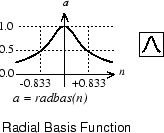Normalized radial basis transfer function

## Graph and Symbol## Syntax

```A = radbasn(N,FP) ```

## Description

`radbasn` is a neural transfer function. Transfer functions calculate a layer’s output from its net input. This function is equivalent to radbas, except that output vectors are normalized by dividing by the sum of the pre-normalized values.

`A = radbasn(N,FP)` takes one or two inputs,

 `N` `S`-by-`Q` matrix of net input (column) vectors `FP` Struct of function parameters (ignored)

and returns `A`, an `S`-by-`Q` matrix of the radial basis function applied to each element of `N`.

## Examples

Here six random 3-element vectors are passed through the radial basis transform and normalized.

```n = rand(3,6) a = radbasn(n) ```

Assign this transfer function to layer `i` of a network.

```net.layers{i}.transferFcn = 'radbasn'; ```

## Algorithms

```a = radbasn(n) = exp(-n^2) / sum(exp(-n^2)) ```

## Version History

Introduced in R2010b